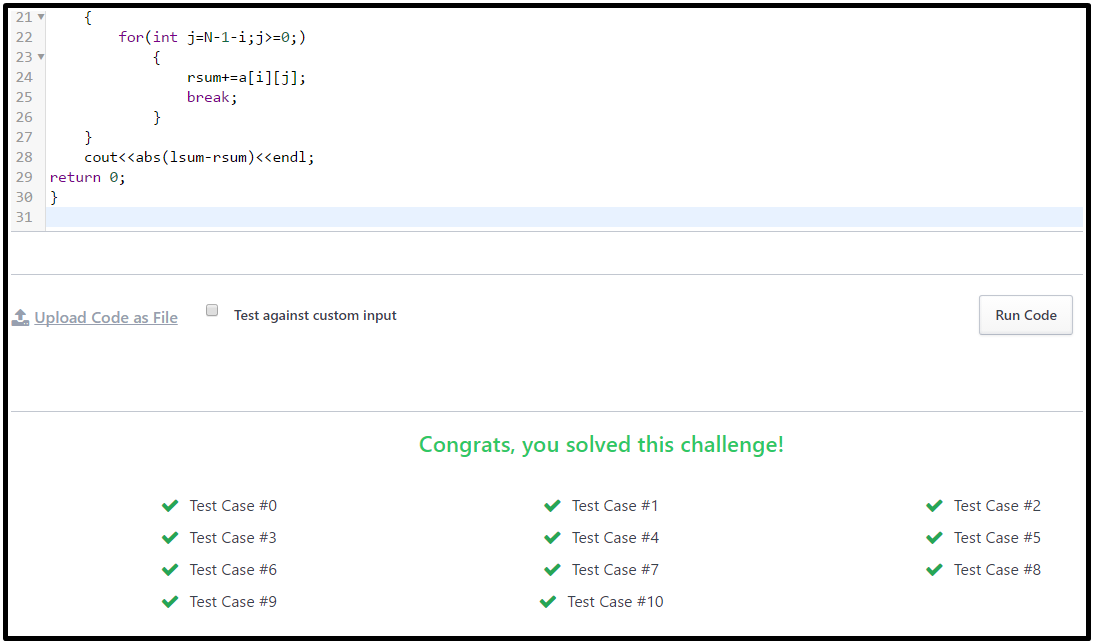# Diagonal Difference Hackerrank Solution in C | C++ Languages

In this article, we are providing Diagonal Difference Hackerrank Solution in C, C++, and Java programming Languages. This programming problem belongs to hackerrank 30 days of code, and we are going to find the Hackerrank Diagonal Difference Solution in C and C++ language. In this programming challenge. We have to find the Diagonal Difference of an N*N matrix or a square matrix. So why we are waiting, let's do it firstly, we have to find the right diagonal sum of a matrix and left a diagonal sum of magic, then we will find out the Diagonal Difference of a matrix. In this matrix, we already know that our matrix is an N*N shape.

## Diagonal Difference Hackerrank- Table of Content

1. Function description
2. Input Format
3. Constraints
4. Output Format
5. Sample Input
6. Sample Output
7. Explanation Diagonal Difference Hackerrank Solution
8. The logic of Diagonal Difference
9. Diagonal Difference Hackerrank Solution in C
10. Diagonal Difference Hackerrank Solution in C++
11. And more...

Given a square matrix, calculate the absolute difference between the sums of its diagonals.

For example, the square matrix arr is shown below:

1 2 3
4 5 6
9 8 9

The left-to-right diagonal = 1 + 5 + 9 = 15 . The right to left diagonal = 3 + 5 + 9 = 17 . Their absolute difference is |15-17| = 2.

### Function description

Complete the Diagonaldifference function in the editor below. It must return an integer representing the absolute diagonal difference.

diagonalDifference takes the following parameter:

arr: an array of integers.

### Input Format

The first line contains a single integer, n, the number of rows and columns in the matrix arr. Each of the next n lines describes a row, arr[i], and consists of n space-separated integers arr[i][j].

### Constraints

-100<=arr[i][j]<=100

### Output Format

Print the absolute difference between the sums of the matrix's two diagonals as a single integer.

3
11 2 4
4 5 6
10 8 -12

15

### Explanation Diagonal Difference Hackerrank Solution Sample

The primary diagonal is:

Sum across the primary diagonal: 11 + 5 - 12 = 4

The secondary diagonal is

Sum across the secondary diagonal: 4 + 5 + 10 = 19

Difference: |4 - 19| = 15

Now the last step is to find the difference between the sum of diagonal, so add first diagonal and second diagonal after that mod the difference so | 4 - 19| = 15. Hence we got our solution.

Note: |x| is the absolute value of x

Check This- Hackerrank 30 days of code Solution.

## Diagonal Difference Hackerrank Solution Logic

So the logic is straightforward in this diagonal difference hackerrank problem. We have to find the diagonal sum of the matrix, and after seeing the total amount. We have to find out the difference between both diagonal sums. If the difference of both diagonal matrices is negative, then find the Mod or, in the end, print the output. Get the Hackerrank Diagonal Difference Solution in C language See the above logic solution with an example in the explanation.

## Diagonal Difference Hackerrank Solution in C

`#include <math.h>#include <stdio.h>#include <string.h>#include <stdlib.h>#include <assert.h>#include <limits.h>#include <stdbool.h>int main(){     int n, j;    int i=0,RightDiagonalSum=0,LeftDiagonalSum=0, firstarray, secondarray;     scanf("%d",&n);    int a[n][n];     for(int firstarray = 0; firstarray < n; firstarray++) {       for(int secondarray = 0; secondarray < n; secondarray++)    {                   scanf("%d",&a[firstarray][secondarray]);       }    }   while(i<n)  {    RightDiagonalSum=RightDiagonalSum+a[i][i];    i++;  }     j=n-1,i=0;      while(i<n)  {    LeftDiagonalSum=LeftDiagonalSum+a[i][j];    i++;    j--;  }     printf("%d",abs(RightDiagonalSum-LeftDiagonalSum));    return 0;}`

So as we already know, what is the logic,, so without wasting time, let's just implement the above logic in our diagonal difference hackerrank problem with an example? Take a MATRIX with the same number of rows and columns. Below is the Hackerrank Diagonal Difference Solution in C++ Programming language.

## Diagonal Difference Hackerrank Solution in C++

`#include <iostream>using namespace std;int main() {  int N, LeftDiagonalSum = 0, RightDiagonal = 0;  cin >> N;  int a[N][N];  for (int i = 0; i < N; i++)   {    for (int j = 0; j < N; j++)     {      cin >> a[i][j];      if (i == j)    {        LeftDiagonalSum = LeftDiagonalSum + a[i][j];      }    }  }    for (int i = 0; i < N; i++)   {    for (int j = N - 1 - i; j >= 0;)     {      RightDiagonal = RightDiagonal + a[i][j];      break;    }  }  cout << abs(LeftDiagonalSum - RightDiagonal) << endl;  return 0;}`

### Diagonal Difference Hackerrank Solution Output### Similar to Hackerrank Diagonal Difference Solution

Previous Post
Next Post

#### post written by: Ghanendra Yadav

Hi, I’m Ghanendra Yadav, SEO Expert, Professional Blogger, Programmer, and UI Developer. Get a Solution of More Than 500+ Programming Problems, and Practice All Programs in C, C++, and Java Languages. Get a Competitive Website Solution also Ie. Hackerrank Solutions and Geeksforgeeks Solutions. If You Are Interested to Learn a C Programming Language and You Don't Have Experience in Any Programming, You Should Start with a C Programming Language, Read: List of Format Specifiers in C.